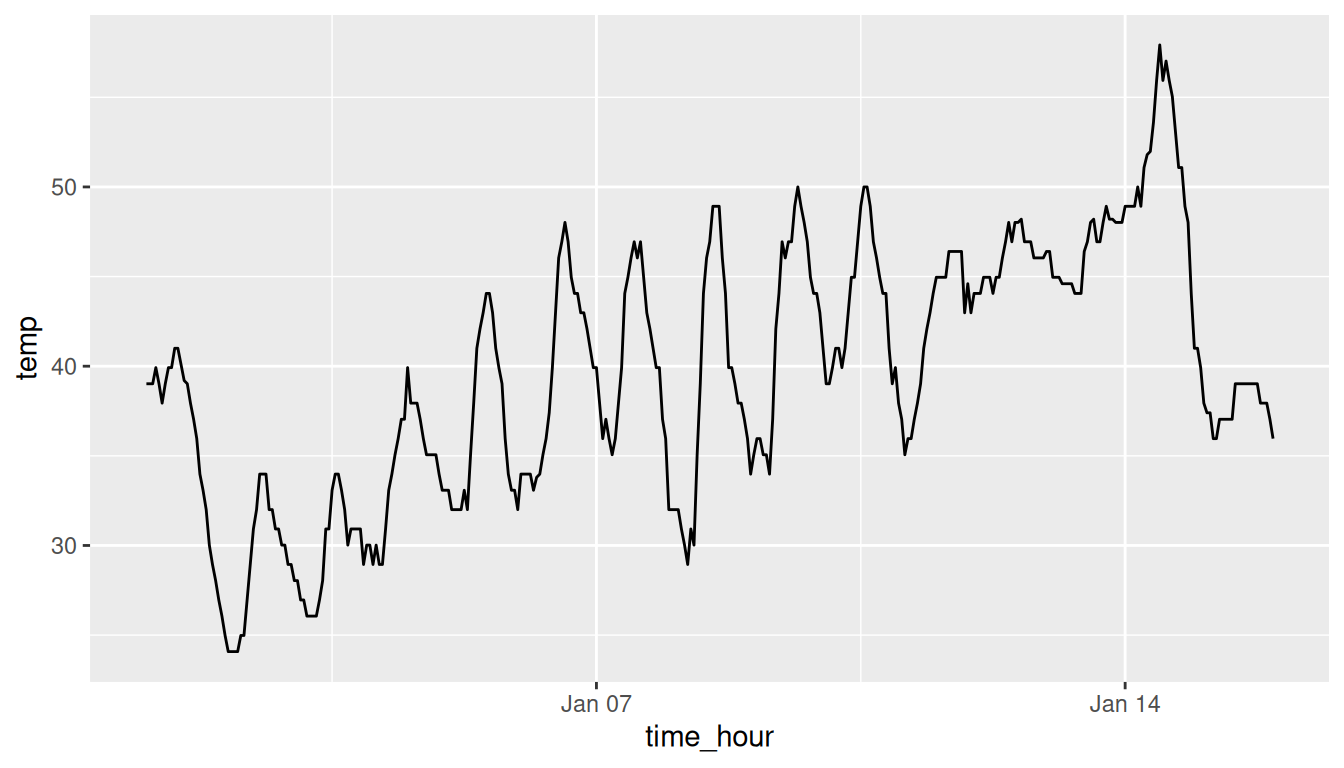## 2.4 5NG#2: Linegraphs

The next of the five named graphs are linegraphs. Linegraphs show the relationship between two numerical variables when the variable on the x-axis, also called the explanatory variable, is of a sequential nature. In other words, there is an inherent ordering to the variable.

The most common examples of linegraphs have some notion of time on the x-axis: hours, days, weeks, years, etc. Since time is sequential, we connect consecutive observations of the variable on the y-axis with a line. Linegraphs that have some notion of time on the x-axis are also called time series plots. Let’s illustrate linegraphs using another dataset in the nycflights13 package: the weather data frame.

Let’s explore the weather data frame by running View(weather) and glimpse(weather). Furthermore let’s read the associated help file by running ?weather to bring up the help file.

Observe that there is a variable called temp of hourly temperature recordings in Fahrenheit at weather stations near all three major airports in New York City: Newark (origin code EWR), John F. Kennedy International (JFK), and LaGuardia (LGA). However, instead of considering hourly temperatures for all days in 2013 for all three airports, for simplicity let’s only consider hourly temperatures at Newark airport for the first 15 days in January.

Recall in Section 2.3, we used the filter() function to only choose the subset of rows of flights corresponding to Alaska Airlines flights. We similarly use filter() here, but by using the & operator we only choose the subset of rows of weather where the origin is "EWR", the month is January, and the day is between 1 and 15. Recall we performed a similar task in Section 2.3 when creating the alaska_flights data frame of only Alaska Airlines flights, a topic we’ll explore more in Chapter 3 on data wrangling.

early_january_weather <- weather %>%
filter(origin == "EWR" & month == 1 & day <= 15)

Learning check

(LC2.9) Take a look at both the weather and early_january_weather data frames by running View(weather) and View(early_january_weather). In what respect do these data frames differ?

(LC2.10) View() the flights data frame again. Why does the time_hour variable uniquely identify the hour of the measurement, whereas the hour variable does not?

### 2.4.1 Linegraphs via geom_line

Let’s create a time series plot of the hourly temperatures saved in the early_january_weather data frame by using geom_line() to create a linegraph, instead of using geom_point() like we used previously to create scatterplots:

ggplot(data = early_january_weather,
mapping = aes(x = time_hour, y = temp)) +
geom_line()FIGURE 2.7: Hourly temperature in Newark for January 1-15, 2013.

Much as with the ggplot() code that created the scatterplot of departure and arrival delays for Alaska Airlines flights in Figure 2.2, let’s break down this code piece-by-piece in terms of the grammar of graphics:

Within the ggplot() function call, we specify two of the components of the grammar of graphics as arguments:

1. The data to be the early_january_weather data frame by setting data = early_january_weather.
2. The aesthetic mapping by setting mapping = aes(x = time_hour, y = temp). Specifically, the variable time_hour maps to the x position aesthetic, while the variable temp maps to the y position aesthetic.

We add a layer to the ggplot() function call using the + sign. The layer in question specifies the third component of the grammar: the geometric object in question. In this case, the geometric object is a line set by specifying geom_line().

Learning check

(LC2.11) Why should linegraphs be avoided when there is not a clear ordering of the horizontal axis?

(LC2.12) Why are linegraphs frequently used when time is the explanatory variable on the x-axis?

(LC2.13) Plot a time series of a variable other than temp for Newark Airport in the first 15 days of January 2013.

### 2.4.2 Summary

Linegraphs, just like scatterplots, display the relationship between two numerical variables. However, it is preferred to use linegraphs over scatterplots when the variable on the x-axis (i.e., the explanatory variable) has an inherent ordering, such as some notion of time.